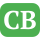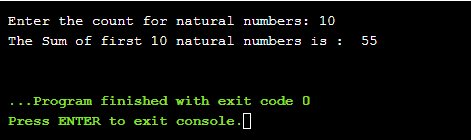CppBuzz.com

# C program to compute sum of first n natural number using while loop

This C program takes count of natural numbers from user and then calculates the sum using while loop.

``````#include<stdio.h>

int main()
{
int no, i,sum = 0;

printf("Enter the count for natural numbers: ");
scanf("%d", &no);

i=1;
while(i<=no) {
sum = i + sum ;
i++ ;
}

printf("The Sum of first %d natural numbers is :  %d\n", no, sum);
return 0;
}
```
```

## Sum of first 10 natural numbers